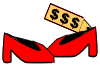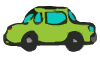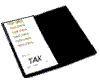# Sales Tax

## Learn How To Determine Sales Tax With Step By Step Problems And Solutions. Use the Interactive Exercises to Assess Your Understanding.Example 1: Mr. Smith and his family came to the USA for a visit from Melbourne, Australia. After purchasing \$32.00 in clothing at a department store, he was presented with a bill for \$34.36. Why did the department store charge him the additional \$2.36? How did the department store compute this amount? What amount should Mr. Smith pay?

Analysis: The department store was adding sales tax to the bill. Sales tax is a tax on goods and services purchased and is normally a certain percentage added to the buyer's cost.

Solution: 2.36 ÷ \$32.00 = 0.0735. Sales tax was charged by the department store at a rate of 7.375%.

Answer: Mr. Smith should pay the department store \$32.00 plus \$2.36 in sales tax for a total bill of \$34.36.

Sales tax rates vary from state to state. Retail sales tax rates are set by the individual states in the United States and vary from 0 to 9% of the sales price. Sales tax is usually the responsibility of the merchant to collect and submit to the state, and stated separately (or implicitly added at the time of the sale) to consumers. Let's look at some more examples of sales tax.Example 2: If the sales tax rate is 7.25% in California, then how much would you pay in Los Angeles for a pair of shoes that cost \$39.00?

Solution: (\$39.00)*(0.0725) = \$2.8275. Round to the nearest cent, the sales tax is \$2.83.

Answer: You would pay \$39.00 plus \$2.83 in sales tax for a total of \$41.83.Example 3: A roll of plastic electrical tape costs \$2.79. If the sales tax rate is 5%, then how much sales tax is being charge? What is the total cost for the tape including tax?

Solution: (\$2.79)*(0.05) = \$0.1395. Rounded to the nearest cent the sales tax is \$0.14.

Answer: The sales tax is \$0.14 and the total cost is \$2.79 + \$0.14 = \$2.93.Example 4: The price of a new car is \$29,990. If the sales tax rate is 6.5%, then how much sales tax is being charged? What is the total cost for the car including tax?

Solution: (\$29990)*(0.065) = \$1949.35.

Answer: The sales tax is \$1949.35 and the total cost is \$29,990.00 + \$1949.35 = \$31,939.35.

As you can see, for small purchases, sales tax can be a nuisance; whereas for large purchases, sales tax can be a significant amount. Let's look at an example in which the sales tax rate is unknown.Example 5: If your restaurant food was \$17.00 and the total bill was \$17.68, then what is the sale tax rate being charged?

Analysis: Sales tax is the difference between the amount of the total bill and the price of the item. If we divide the sales tax by the price of the item, we get the sales tax rate.

Solution: \$17.68 - \$17.00 = \$0.68 and (\$0.68) ÷ (\$17.00) = 0.04.

Answer: The sales tax rate is 4%.

Summary: Sales tax is a tax on goods and services purchased and is normally a percentage added to the buyer's cost.

### Exercises

Directions: Each problem below involves sales tax. Solve each problem below by entering a dollar amount with cents. Round to the nearest cent when necessary. Do not enter a comma in your answer. For each exercise below, click once in the ANSWER BOX, type in your answer and then click ENTER. After you click ENTER, a message will appear in the RESULTS BOX to indicate whether your answer is correct or incorrect. To start over, click CLEAR.

 1 If the sales tax rate is 7.375% in New York State, then how much sales tax would you pay in Albany for a \$34 pair of pants? ANSWER BOX:  \$  RESULTS BOX:
 2 If the sales tax rate is 5.25% in New Jersey, then what is the total price including tax for a \$25.00 piece of jewelry bought in Trenton? ANSWER BOX:  \$  RESULTS BOX:
 3 Amy bought a dress marked \$42 and then paid the store clerk a total of \$43.47. What was the sales tax rate? (Enter your answer as a percent.) ANSWER BOX:  %  RESULTS BOX:
 4 If the sales tax rate is 8.375% in New York City, then how much sales tax would you pay for a new car in Manhattan that costs \$31,500? ANSWER BOX:  \$  RESULTS BOX:
 5 If the sales tax rate is 7.25% in California, then how much tax should a merchant charge in San Francisco for the sale of a \$15 scarf? ANSWER BOX:  \$  RESULTS BOX: